Home    |    Teacher    |    Parents    |    Glossary    |    About UsEmail this page to a friendResources· Cool Tools · Formulas & Tables · References · Test Preparation · Study Tips · Wonders of MathSearchArea Formulas (Math | Geometry | Area Formulas)

(pi =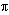= 3.141592...)

### Be careful!! Units count. Use the same units for all measurements. Examples

square = a 2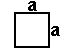rectangle = ab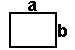parallelogram = bh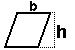trapezoid = h/2 (b1 + b2)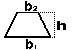circle = pi r 2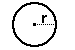ellipse = pi r1 r2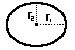triangle =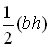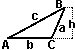one half times the base length times the height of the triangle

 equilateral triangle =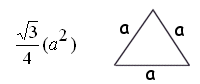triangle given SAS (two sides and the opposite angle)
= (1/2) a b sin C

triangle given a,b,c =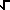[s(s-a)(s-b)(s-c)] when s = (a+b+c)/2 (Heron's formula)

regular polygon = (1/2) n sin(360°/n) S2
when n = # of sides and S = length from center to a cornerUnits

Area is measured in "square" units. The area of a figure is the number of squares required to cover it completely, like tiles on a floor.

Area of a square = side times side. Since each side of a square is the same, it can simply be the length of one side squared.

If a square has one side of 4 inches, the area would be 4 inches times 4 inches, or 16 square inches. (Square inches can also be written in2.)

Be sure to use the same units for all measurements. You cannot multiply feet times inches, it doesn't make a square measurement.

The area of a rectangle is the length on the side times the width. If the width is 4 inches and the length is 6 feet, what is the area?

NOT CORRECT .... 4 times 6 = 24

CORRECT.... 4 inches is the same as 1/3 feet. Area is 1/3 feet times 6 feet = 2 square feet. (or 2 sq. ft., or 2 ft2).